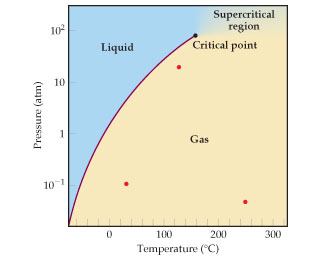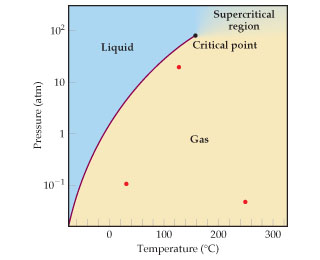Chemistry Practice Problems Equilibrium Expressions Practice Problems Solution: The phase diagram for SO2 is shown here.Calculate ...

🤓 Based on our data, we think this question is relevant for Professor Borger's class at UNC.

# Solution: The phase diagram for SO2 is shown here.Calculate the equilibrium constant for this reaction at 100 oC.

###### Problem

The phase diagram for SO2 is shown here.Calculate the equilibrium constant for this reaction at 100 oC.

###### Solution

We are being asked the equilibrium constant for the reaction at 100 oC.Recall: The equilibrium constant K is a ratio of products to reactants at a given temperature. It only deals with gaseous or aqueous compoundsView Complete Written Solution

Equilibrium Expressions

Equilibrium Expressions

#### Q. The following diagram represents a reaction shown going to completion. Each molecule in the diagram represents 0.1 mol, and the volume of the box is 1...

Solved • Tue Dec 11 2018 13:37:50 GMT-0500 (EST)

Equilibrium Expressions

#### Q. The equilibrium2NO (g) + Cl2 (g) 2NOCl (g)is established at 500 K. An equilibrium mixture of the three gases has partial pressures of 9.50×10−2 atm , ...

Solved • Tue Dec 11 2018 13:37:39 GMT-0500 (EST)

Equilibrium Expressions

#### Q. Consider the reaction A(g) ⇌ B(g). The images shown here illustrate equilibrium mixtures of A (red) and g (black) at three different temperatures.At w...

Solved • Tue Dec 11 2018 13:37:37 GMT-0500 (EST)

Equilibrium Expressions

#### Q. Consider the reaction: A(g) ⇌ 2B(g). The graph plots the concentrations of A and B as a function of time at a constant temperature.What is the equilib...

Solved • Tue Dec 11 2018 13:37:37 GMT-0500 (EST)